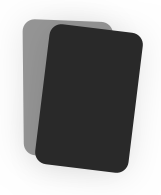# FI 3300 FINAL EXAM

0.0(0) Reviews
Duplicate
Report Flashcard set

#### Spaced Repetition

Scientifically backed study method#### Flashcards

Review terms and definitions#### Learn

Study with MC, T/F, and other questions#### Practice Test

Take a test on your terms and definitions## Tags

21 Terms
😃 Not studied yet (21)
Assets
Current Assets - cash - net accounts receivable (gross - allowance for doubtful accounts) - Inventories Long term assets - Net fixed assets (gross fixed assets - less accumulated depreciation) = total assets
Liabilities
Current liabilities - notes payable - accounts payable - accrued expenses - current portion long term debt Long term liabilities - long term debt (less current portion) = total liabilites
Stockholder's equity
Common stock Preferred stock Additional paid in capital Retained earnings = total equity
Net accounts receivable
Gross accounts receivable - allowance for doubtful accounts
Net fixed assets
net fixed assets = gross fixed assets - accumulated depreciation
Annual addition to RE = Net income - dividend payout
Year end Retained earnings
year end RE = beginning RE + annual addition to RE
Cost of goods sold
COGS = Beginning inv + material purchases - ending inv
Net sales
Gross sales - sales returns & allowances
Debt to equity ratio
total liabilities / equity
Income statement
Net sales - COGS = Gross profit - operating expenses (less depreciation if retail biz) = Operating profit (EBIT) - interest expense = EBT (earnings before taxes) - taxes = Net Income
OpEx
1. Management salaries 2. R&D 3. Lease payments 4. Repairs & Maintenance 5. General and admin expenses
Common size income statement
Income statement item / net sales
Common size balance sheet
Balance sheet item / total assets
Tax equivalent yield
= tax free yield / (1 - tax rate)
Required rate of return
R(equity) = R(risk free) + B(Rm - Rf)M